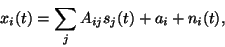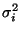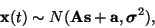Next: Neural network interpretation of Up: LINEAR FACTOR ANALYSIS AND Previous: LINEAR FACTOR ANALYSIS AND

## Linear Gaussian factor analysis model

According to the model used in ordinary factor analysis, the observations xi are weighted sums of underlying latent variables. In other words, the dependences between the different components in an observation vector are assumed to be caused by common factors. For consistency with the rest of the thesis, the factors will be denoted by s, although according to the usual convention they would be denoted by f.

The linear summation model is quite simple and it is reasonable to assume there are inaccuracies in the model and many other causes for the observations besides the factors included in the model. The effect of the inaccuracies and other causes is summarised by Gaussian noise n. In anticipation of the dynamic model, the observations are indexed by t referring to time, although in the usual factor analysis model, observations at different time instants are assumed to be independent of each other and the observations therefore need not form a sequence in time.

The linear factor analysis model can be written as(25)

where i indexes different components of the observation vector, jindexes different factors and Aij are the weightings of the factors, also known as factor loadings. The factors s and noise nare assumed to have zero mean. The bias in x is assumed to be caused by a. This is called a generative model since it explicitly gives the hypothesis about how the observations were generated.

The model can be written in a vector form as

 x(t) = A s(t) + a + n(t). (26)

Here x, s, a and n are vectors and A is a matrix. This more compact vector form is used by default throughout the thesis.

If the variances of the Gaussian noise terms ni(t) are denoted by, the probability which the model gives for the observation xi(t) can be written as(27)

This can also be written simply as(28)

where the vectorcontains the variances. This notation is used for emphasising that the covariance matrix of x(t) is diagonal, i.e., the noise on different components is assumed to be independent.

For mathematical convenience, the factors are assumed to have Gaussian distributions in the standard factor analysis model. Recall that the Gaussian distribution emerges if a large number of independent variables are summed linearly. Effectively the Gaussian model for factors then means that the factors are themselves assumed to be caused by various other factors. For many purposes this may be a suitable simplification but it means that the Gaussian factor analysis model is not able to reveal the original independent causes of the observations even if there would be some. Mathematically, this manifests itself in the fact that a multivariate Gaussian distribution with equal variances for all factors is spherically symmetric. Any rotation of the variables will leave the distribution unchanged, and therefore there is a rotational indeterminacy in the model. If the variances of the factors differ, the indeterminacy still exists but the corresponding rotation is non-orthogonal.

From a practical point of view this means that the Gaussian model is able to capture only the second order correlation structure of the components of the observation vectors. Additional criteria can be used to fix the rotation of the matrix A, but it is usually not reasonable to directly interpret the factors as the original independent causes of the observations.Next: Neural network interpretation of Up: LINEAR FACTOR ANALYSIS AND Previous: LINEAR FACTOR ANALYSIS AND
Harri Valpola
2000-10-31